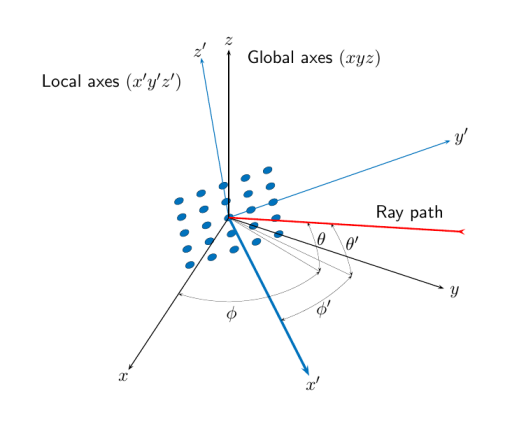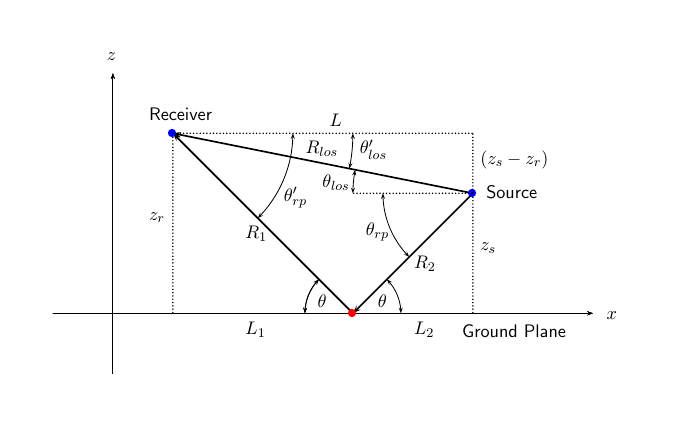# rangeangle

Range and angle calculation

## Syntax

``````[rng,ang] = rangeangle(pos)``````
``````[rng,ang] = rangeangle(pos,refpos)``````
``````[rng,ang] = rangeangle(pos,refpos,refaxes)``````
``````[rng,ang] = rangeangle(___,model)``````

## Description

The function `rangeangle` determines the propagation path length and path direction of a signal from a source point or set of source points to a reference point. The function supports two propagation models – the free space model and the two-ray model. The free space model is a single line-of-sight path from a source point to a reference point. The two-ray multipath model generates two paths. The first path follows the free-space path. The second path is a reflected path off a boundary plane at z = 0. Path directions are defined with respect to either the global coordinate system at the reference point or a local coordinate system at the reference point. Distances and angles at the reference point do not depend upon which direction the signal is travelling along the path.

example

``````[rng,ang] = rangeangle(pos)``` returns the propagation path length, `rng`, and direction angles, `ang`, of a signal path from a source point or set of source points, `pos`, to the origin of the global coordinate system. The direction angles are the azimuth and elevation with respect to the global coordinate axes at the origin. Signals follow a line-of-sight path from the source point to the origin. The line-of-sight path corresponds to the geometric straight line between the points.```

example

``````[rng,ang] = rangeangle(pos,refpos)``` also specifies a reference point or set of reference points, `refpos`. `rng` now contains the propagation path length from the source points to the reference points. The direction angles are the azimuth and elevation with respect to the global coordinate axes at the reference points. You can specify multiple points and multiple reference points.```

example

``````[rng,ang] = rangeangle(pos,refpos,refaxes)``` also specifies local coordinate system axes, `refaxes`, at the reference points. Direction angles are the azimuth and elevation with respect to the local coordinate axes centered at `refpos`.```

``````[rng,ang] = rangeangle(___,model)```, also specifies a propagation model. When `model` is set to `'freespace'`, the signal propagates along a line-of-sight path from source point to reception point. When `model` is set to `'two-ray'`, the signal propagates along two paths from source point to reception point. The first path is the line-of-sight path. The second path is the reflecting path. In this case, the function returns the distances and angles for two paths for each source point and corresponding reference point.```

## Input Arguments

 `pos` Source point position, specified as a real-valued 3-by-1 vector or a real-valued 3-by-N matrix. A matrix represents multiple source points. The columns contain the Cartesian coordinates of N points in the form `[x;y;z]`. When `pos` is a 3-by-N matrix, you must specify `refpos` as a 3-by-N matrix for N reference positions. If all the reference points are identical, you can specify `refpos` by a single 3-by-1 vector. Position units are meters. `refpos` Reference point position, specified as a real-valued 3-by-1 vector or a real-valued 3-by-N matrix. A matrix represents multiple reference points. The columns contain the Cartesian coordinates of N points ins the form `[x;y;z]`. When `refpos` is a 3-by-N matrix, you must specify `pos` as a 3-by-N matrix for N source positions. If all the source points are identical, you can specify `pos` by a single 3-by-1 vector. Position units are meters. Default: `[0;0;0]` `refaxes` Local coordinate system axes, specified as a real-valued 3-by-3 matrix or a 3-by-3-by-N array. For an array, each page corresponds to a local coordinate axes at each reference point. The columns in `refaxes` specify the direction of the coordinate axes for the local coordinate system in Cartesian coordinates. N must match the number of columns in `pos` or `refpos` when these dimensions are greater than one. Default: `[1 0 0;0 1 0;0 0 1]` `model` Propagation model, specified as `'freespace'` or `'two-ray'`. Choosing `'freespace'` invokes the free space propagation model. Choosing `'two-ray'` invokes the two-ray propagation model. Default: `'freespace'`

## Output Arguments

 `rng` Propagation range, returned as a real-valued 1-by-N vector or real-valued 1-by-2N vector. When `model` is set to `'freespace'`, the size of `rng` is 1-by-N. The propagation range is the length of the direct path from the position defined in `pos` to the corresponding reference position defined in `refpos`. When `model` is set to `'two-ray'`, `rng` contains the ranges for the direct path and the reflected path. Alternate columns of `rng` refer to the line-of-sight path and reflected path, respectively for the same source-reference point pair. Position units are meters. `ang` Azimuth and elevation angles, returned as a 2-by-N matrix or 2-by-2N matrix. Each column represents a direction angle in the form `[azimuth;elevation]`. When `model` is set to `'freespace'`, `ang` is a 2-by-N matrix and represents the angle of the path from a source point to a reference point. When `model` is set to `'two-ray'`, `ang` is a 2-by-2N matrix. Alternate columns of `ang` refer to the line-of-sight path and reflected path, respectively. Angle units are in degrees.

## Examples

collapse all

Compute the range and angle of a target located at (1000,2000,50) meters from the origin.

```TargetLoc = [1000;2000;50]; [tgtrng,tgtang] = rangeangle(TargetLoc)```
```tgtrng = 2.2366e+03 ```
```tgtang = 2×1 63.4349 1.2810 ```

Compute the range and angle of a target located at (1000,2000,50) meters with respect to a local origin at (100,100,10) meters.

```TargetLoc = [1000;2000;50]; Origin = [100;100;10]; [tgtrng,tgtang] = rangeangle(TargetLoc,Origin)```
```tgtrng = 2.1028e+03 ```
```tgtang = 2×1 64.6538 1.0900 ```

Compute the range and angle of a target located at (1000,2000,50) meters but with respect to a local coordinate system origin at (100,100,10) meters. Choose a local coordinate reference frame that is rotated about the z-axis by 45° from the global coordinate axes.

```targetpos = [1000;2000;50]; origin = [100;100;10]; refaxes = [1/sqrt(2) -1/sqrt(2) 0; 1/sqrt(2) 1/sqrt(2) 0; 0 0 1]; [tgtrng,tgtang] = rangeangle(targetpos,origin,refaxes)```
```tgtrng = 2.1028e+03 ```
```tgtang = 2×1 19.6538 1.0900 ```

collapse all

### Angles in Local and Global Coordinate Systems

The `rangeangle` function returns the path distance and path angles in either the global or local coordinate systems. By default, the `rangeangle` function determines the angle a signal path makes with respect to global coordinates. If you add the `refaxes` argument, you can compute the angles with respect to local coordinates. As an illustration, this figure shows a 5-by-5 uniform rectangular array (URA) rotated from the global coordinates (xyz) using `refaxes`. The x' axis of the local coordinate system (x'y'z') is aligned with the main axis of the array and moves as the array moves. The path length is independent of orientation. The global coordinate system defines the azimuth and elevations angles (Φ,θ) and the local coordinate system defines the azimuth and elevations angles (Φ',θ').

Local and Global Coordinate Axes### Free Space Propagation Model

The free-space signal propagation model states that a signal propagating from one point to another in a homogeneous, isotropic medium travels in a straight line, called the line-of-sight or direct path. The straight line is defined by the geometric vector from the radiation source to the destination.

### Two-Ray Propagation Model

A two-ray propagation channel is the next step up in complexity from a free-space channel and is the simplest case of a multipath propagation environment. The free-space channel models a straight-line line-of-sight path from point 1 to point 2. In a two-ray channel, the medium is specified as a homogeneous, isotropic medium with a reflecting planar boundary. The boundary is always set at z = 0. There are at most two rays propagating from point 1 to point 2. The first ray path propagates along the same line-of-sight path as in the free-space channel. The line-of-sight path is often called the direct path. The second ray reflects off the boundary before propagating to point 2. According to the Law of Reflection , the angle of reflection equals the angle of incidence. In short-range simulations such as cellular communications systems and automotive radars, you can assume that the reflecting surface, the ground or ocean surface, is flat.

The figure illustrates two propagation paths. From the source position, ss, and the receiver position, sr, you can compute the arrival angles of both paths, θ′los and θ′rp. The arrival angles are the elevation and azimuth angles of the arriving radiation with respect to a local coordinate system. In this case, the local coordinate system coincides with the global coordinate system. You can also compute the transmitting angles, θlos and θrp. In the global coordinates, the angle of reflection at the boundary is the same as the angles θrp and θ′rp. The reflection angle is important to know when you use angle-dependent reflection-loss data. You can determine the reflection angle by using the `rangeangle` (Phased Array System Toolbox) function and setting the reference axes to the global coordinate system. The total path length for the line-of-sight path is shown in the figure by Rlos which is equal to the geometric distance between source and receiver. The total path length for the reflected path is Rrp= R1 + R2. The quantity L is the ground range between source and receiver.You can easily derive exact formulas for path lengths and angles in terms of the ground range and object heights in the global coordinate system.

`$\begin{array}{l}\stackrel{\to }{R}={\stackrel{\to }{x}}_{s}-{\stackrel{\to }{x}}_{r}\\ {R}_{los}=|\stackrel{\to }{R}|=\sqrt{{\left({z}_{r}-{z}_{s}\right)}^{2}+{L}^{2}}\\ {R}_{1}=\frac{{z}_{r}}{{z}_{r}+{z}_{z}}\sqrt{{\left({z}_{r}+{z}_{s}\right)}^{2}+{L}^{2}}\\ {R}_{2}=\frac{{z}_{s}}{{z}_{s}+{z}_{r}}\sqrt{{\left({z}_{r}+{z}_{s}\right)}^{2}+{L}^{2}}\\ {R}_{rp}={R}_{1}+{R}_{2}=\sqrt{{\left({z}_{r}+{z}_{s}\right)}^{2}+{L}^{2}}\\ \mathrm{tan}{\theta }_{los}=\frac{\left({z}_{s}-{z}_{r}\right)}{L}\\ \mathrm{tan}{\theta }_{rp}=-\frac{\left({z}_{s}+{z}_{r}\right)}{L}\\ {{\theta }^{\prime }}_{los}=-{\theta }_{los}\\ {{\theta }^{\prime }}_{rp}={\theta }_{rp}\end{array}$`

## Version History

Introduced in R2019b